# Why Data Types in C++

## Why Data Types in C++?

In this article, I am going to discuss Why do we need Data Types in C++. Please read our previous article where we discussed how to write the basic C++ Program. So, at the end of this article, you will understand What is Data Type and Why we need Data Types in C++.

##### Why do we need Data Types in C++?

Let us first understand why we need data types. Program is a set of two ingredients. That is data and instruction or operations that are performed on the data. So, Program or instruction always acts on Data. Let’s explain this in detail. Usually, the main memory is divided into the code section, stack, heap section as shown in the below image.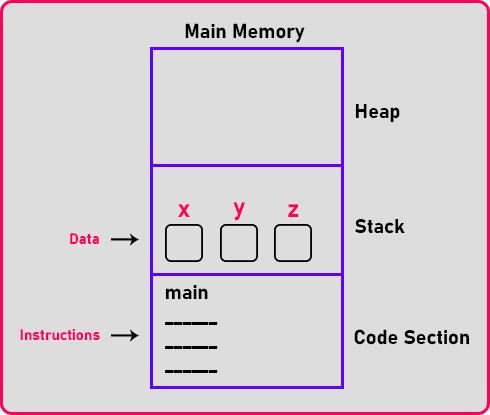There are three sections that are heap, stack, and code section. Whenever we execute the program all the instruction is loaded into the code section and data is loaded into the stack section/heap section depending on where exactly we need to store the data but usually, data is stored in the stack section.

So let us understand how we work in daily life. In our daily life, we use the data in various places like for example the amount of bill, price of some item or your roll number or marks, house number, your name. Various things are there, this is nothing but data.

Now let’s talk about data types. As I said earlier program acts on data but program logic depends on what type of data we are manipulating or handling. Usually, Data can be of two types. Numeric data and character or alphabets data. If you are interested in performing arithmetic operations then we usually use and manipulate numeric data. So, we will categorize the data, into two types.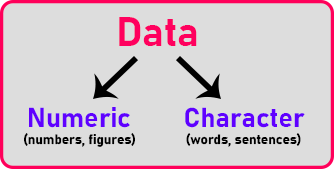One is numeric data that is a set of numbers or figures. And other we use character type data. Character types are words or sentences or names. We can also call this an alphabetic type of data.

Example of numeric data:10,10.5,01010,0X10 etc. If you are interested in sorting employee names, addresses, etc. then we usually use character or string data. Example: ‘A’, “Ashwath”, “Ash26”, etc.

So, data is either numeric or alphabetic or you can say character and sometimes we use mix often numeric and alphabetic like if I’m writing a door number, i.e. 1145-A, that’s a door number. Let us call it alphabetic otherwise, we can also say alpha-numeric.

Let us pick up numeric. If I have a number that is the price for some item, let’s say 5 dollars or a roll number of a student let’s say 31, etc. If you observe this is not having decimal.

So, with this, I can say that in our daily life we use two types of numbers. One is with a decimal and another without a decimal. We don’t usually differentiate them we say it’s a number only but when it comes to computers remember that number has to be represented in binary form.

##### Then how do we represent a decimal?

It’s a problem. So, it has to be handled specially. So, without decimal numbers are different and decimal numbers are different. All electronic devices like computers, your mobile phone, calculator, or any electronic devices with numbers are used to treat these two numbers differently because there is an extra effort required for presenting decimal point numbers. So, we will categorize this number as an integer that is without a decimal. And next is the floating point with the decimal.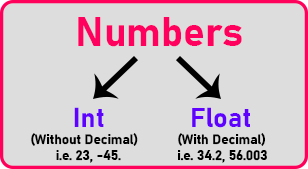The way of representing the decimal number is called Floating Point. This is how, not just C++, all the programming languages like java, C#, Python anything you take they differentiate the data like this and floating-point is a standard. All machine follows the same method for representing a decimal point.

##### Character Data:

Character data can be a name of a person, the name of a place or it may be any word from the dictionary. Actually, the character is just a single letter, but together they are forming it as a string: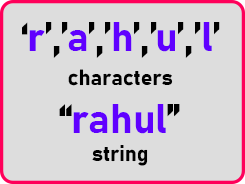So, we call this a string in our program. The string is nothing but a collection of alphabets or characters that are forming a single entity. It may be a name of a person or a word or any word. As we said, these numbers have to be represented in binary then what about the characters? They are also represented in binary.

There are some codes used for representing characters that we will learn when we will discuss characters. This is about different data types we use in daily life. This type of data has to be stored in the memory so that the program can work on that. Memory is defined or represented in terms of bytes, if I take one bite,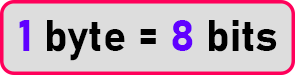1 byte is nothing but a collection of 8 bits: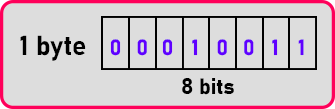Now the question is if you want to store digitized data. Shall we take just one byte? So, in one bite what is the maximum number that we can store? That is minimum is ‘0’. And the max is ‘255’. So, the largest number is 255 we cannot have bigger figures. Then shall we take more than one byte? Yes.

##### Shall we take two bytes or 4 bytes?

How many bytes it would take? And what is the minimum value? What is the maximum value it will store? These are the type of things that we will learn in the upcoming articles. We will give you a detailed explanation of all the data types that are available in C++.

##### Now let us answer why we need Data Types?

We need Data types to inform the Operating System program what is the type of data we are handling based on the type of data it will allocate memory in Bytes in the main memory for the particular Data Types. Let us discuss the data types available in C++.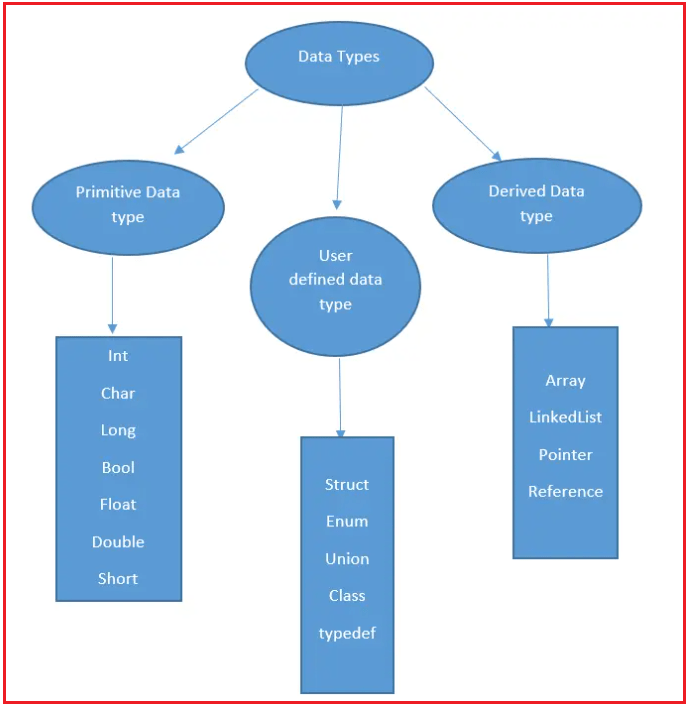The above diagram represents the High-level classification of Data types and each data type will be discussed in detail in our upcoming articles.

In the next article, I am going to discuss Primitive Data Types in C++ with Examples. Here, in this article, I try to explain Why We need Data Types in C++ and I hope you enjoy this Why Data Types in C++ article.